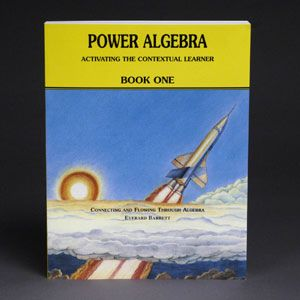Making You An Instant Master Teacher
•Power Algebra Book 1

\$75.00

In spite of your insecurity regarding mathematics, this book can empower you to become a master teacher of algebra for your child's sake. It is carefully written to permit you to perceive and verbalize the connections and flow within algebra.

Introducing Integers, Adding Integers and Three Methods for Subtracting Integers Simplifying Expressions Involving Sums and Differences of Integers and Combining Like Terms Multiplying and Dividing with Integers Multiplying and Dividing with Powers The Positive, Negative and Zero Exponents Multiplication/Division with Monomials and Expressions Involving Negative and Zero Exponents Evaluation of Algebraic Expressions and Order of Operations Adding and Subtracting with Polynomials Multiplying with Polynomials and Factoring by Removing the Highest Common Factor Multiplying with Polynomials and Factoring Trinomials of the Form ax² + bx + c and ax² + bxy + cy² Multiplying with Polynomials and Factoring the Difference of Two Squares Multiplying with Polynomials and Factoring the Sum and Difference of Two Cubes Multiplying with Polynomials and Factoring by Grouping Multiplying with Polynomials and More Advanced Factoring Multiplying with Polynomials and Factoring Completely Dividing with Polynomials and Synthetic Division Binomial Expansion Distinguishing Between Sums and Products – The Real Numbers – Reducing Rational Expressions to Lowest Terms Multiplying and Dividing with Rational Expressions